A rapid look at variational inference with KL divergence

I’ve been spending some time reading up on variational autoencoders (VAEs), which are a paradigm of machine learning that draws some interesting parallels with Bayesian Inference. In order to understand why VAEs worked (aside from just saying neural networks and refusing to think further), I had to get at least a surface level understanding of variational methods.

This information is rephrased from the Stanford Course on Probabilistic Graphical Models here.

Variational inference seeks to cast (often intractable) inference problems as optimization problems. There are some differences between how various fields refer to inference, but in this case inference signifies extracting information from the probabilistic model. This information may be the probability of a given variable after we sum everything else out (marginal inference) or the most likely assignment to the variables in the model (maximum a-posteriori).

Performing variational inference requires an approximating family of distributions,, and an optimization objective,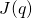. One type of variational inference, called “mean field” uses the product of many univariate distributions as the approximating family. However, there are many other options (for example, VAEs use neural networks as approximating families).

For the optimization objective,, we typically use the KL divergence, which is defined as:The KL divergence is not symmetric (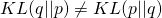) but is always greater than 0 whenand 0 when. This second point is important.

As a specific problem, we show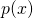to be a joint distribution of many variables, which (for purely pedagogical reasons) is the normalized version of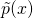.represents the parameters of the distribution, andis the normalizing distribution.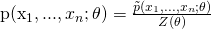From here, we note that we can’t calculateor, but we can still play with our optimization objective.We note that, so it follows that our optimization objective can be expressed as:And re-formed as:The KL divergence betweenand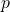is still intractable. HOWEVER, since KL divergences are always greater than or equal to zero, our KL divergence betweenand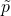(i.e.,) is tractable, and is a lower bound on the probability of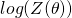. This is called the Variational Lower Bound (VLBO) or more commonly (in my reading) the Evidence Lower Bound (ELBO).

How is this equation useful? It shows up in the mathematics for the variational autoencoder, which I describe in the next post. It also shows up all sorts of other places that I haven’t covered.

This site uses Akismet to reduce spam. Learn how your comment data is processed.